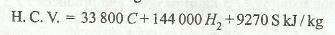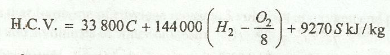# Calorific Value of Fuels

The calorific value (briefly written as CV) or heat value of a solid or liquid fuel may be defined as the amount of heat given out by the complete combustion of 1 kg of fuel. It is expressed in terms of kJ / kg of fuel. The calorific value of gaseous fuels is, however, expressed in terms of kJ / m3 at a specified temperature and pressure.

Following are the two types of the calorific value of fuels:

1. Gross or higher calorific value. The amount of heat obtained by the complete combustion of 1 kg of a fuel, when the products of its combustion are cooled down to the temperature of supplied air (usually taken as 15° C), is called the gross or higher calorific value of fuel. If the chemical analysis of a fuel is available, then the higher calorific value of the fuel is determined by the following formula, known as Dulong's formula:where C, H2 and S represent the mass of carbon, hydrogen and sulphur in 1 kg of fuel, and the numerical values indicate their respective calorific values.

If the fuel contains oxygen (O2) , then it is assumed that the whole amount is combined with hydrogen having mass equal to 1 / 8th of that of oxygen. Therefore, while finding the calorific value of fuel, this amount of hydrogen should be subtracted.2. Net or lower calorific value. When the heat absorbed or carried away by the products of combustion is not recovered (which is the case in actual practice), and the steam formed during combustion is not condensed, then the amount of heat obtained per kg of the fuel is known as net or lower calorific value. It is briefly written as LCV.

If the higher calorific value is known, then the lower calorific value may be obtained by subtracting the amont of heat carried away by products of combustion (especially steam) from HCV.

LCV = HCV - Heat of steam formed during combustion

Notes:

1. The calorific value of solid and liquid fuels may be found by using bomb calorimeter.
2. The calorific value of gaseous and liquid fuels may be found by using any gas calorimeter like Boy's gas calorimeter or Junker's gas calorimeter.